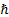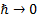/

### Nematrian Website Pages on Quantum Mechanics

Quantum field theory (QFT), the synthesis of quantum mechanics and special relativity, is one of the great achievements of modern physics. It, together with General Relativity, forms the cornerstone of the so-called ‘Standard Model’, i.e. the current scientific understanding of how the universe operates. Many texts explore it in further detail, including Michele Maggiore’s ‘A Modern Introduction to Quantum Field Theory’.

An interesting analogy with modern finance arises from the so-called path integral formulation of quantum mechanics. This formulation is needed to handle some elements of QFT not well handled by other formulations, e.g. non-perturbative elements. In it we calculate the transition probability of a movement from one state to another occurring as the sum over all possible paths which satisfy specified boundary conditions, weighting each path bytimes its ‘action’ in units of. ‘Classical’ mechanics, i.e. the position that rules in the absence of quantum mechanical effects is recovered in a formal sense in the limit as.

An analogy between this formulation of quantum mechanics and derivative pricing theory is mentioned in Malcolm Kemp’s book on Market Consistency. He notes that the ‘market’ price/value of a contingent claim can also be derived by calculating a suitably weighted sum of all of possible paths that the world might take that result in a given outcome.

References

Maggiore, M. (2005). A Modern Introduction to Quantum Field Theory. Oxford University Press